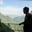Related Tags

properties
binary trees
communitycreator

# What are the properties of binary trees?AKASH BAJWA

### Overview

A binary tree is a tree whose element or node can have a maximum of two children. Let’s look at this special case of trees and learn about their properties.

### Properties of binary trees

The following are the properties of the binary trees:

#### 1. The minimum number of nodes at height h:

In any binary tree, the minimum number of nodes will be one more than the value of height. This is the number of nodes required to construct a tree.

Formula:

1. Height = h.
2. The minimum number of nodes = h+1.
3. If h=3, then nodes will be 3+1= 4.

#### 2. The maximum number of nodes at height h:

The maximum number of nodes that can be inserted in any binary tree of height h will be equal to 2h- 1.

Formula:

1. Height = h.
2. Maximum nodes to inserted = 2h- 1.
3. If h= 3, then 23- 1.
4. 8 -1= 7.

Therefore, the maximum number of nodes to be inserted for the height of h=3 will be 7.

#### 3. Total number of leaf nodes:

The number of leaf nodes in a binary tree is equal to the nodes with degree two, plus one. Say a binary tree has two children. Then the total number of leaf nodes of that binary tree will be one greater than the nodes having two children.

Total number of leaf nodes = Nodes with 2 children + 1

#### Example:

Here, the total number of leaf nodes (no child or a successor) is 3, and the leaf nodes are E, F, and G.

Whereas the nodes with two children are 2. Node A and B have 2 children. Hence, this proves the property.

Binary tree

#### 4. The maximum number of nodes at any level:

In a binary tree, the maximum number of nodes gained by any level is equal to the power of 2 for that level.

In a simpler words, the level is donated by n. Then, maximum nodes of that binary tree will be 2n.

Example:

n = 2 then 22= 4.

Level 2 coverts the nodes (D, E, F, and G).

Binary tree with maximum number of nodes at any level

#### 5. Minimum possible height or levels is equal to Log2(N+1):

This property says that the minimum number of levels or a height of a binary tree is the Log2 of (N+1). Here N represents the maximum number of nodes possessed by a binary tree at the height h.

We have already discussed property 2 along with the example. Let's use that answer to calculate the height h.

1. N = 7 (Using Property#2)
2. Log2 of (N+1) = Log2 of (7+1)
3. Log2(8) = 3

It is equal to the height chosen in the example of property 2.

RELATED TAGS

properties
binary trees
communitycreator

CONTRIBUTORAKASH BAJWA
RELATED COURSES

View all Courses

Keep Exploring

Learn in-demand tech skills in half the time